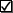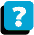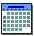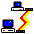Main Module 6 Outline Review for Final Exam

 Learning Objective To provide an overview of the content areas to be covered on the final exam:Descriptive statistics for categorical data (Module 5): Know how to compute probabilities from a cross-classification table, including unconditional (marginal and intersection) and conditional (row and column probabilities). Inferences applied to single proportions For an hypothesis test, be able to set up the null and alternative hypotheses, select an appropriate p-value from computer output, and make the three part test conclusion Be able to interpret a confidence interval from computer output; know how to narrow or widen the margin of error, and know how to compute sample size. Inferences applied to difference between two proportions For an hypothesis test, be able to set up the null and alternative hypotheses, select an appropriate p-value from computer output, and make the three part test conclusion Inferences applied to difference between c proportions using crosss-classification tables and Chi-Square Statistics From computer output, know how to interpret the cross-classification table, test the hypothesis that the proportions are not equal, test the hypothesis that two variables are related, and interpret Cramer's Phi Coefficient.Model Building with Regression Analysis (Modules 2 and 3) On the front end: identify experimental unit (object, person, or thing upon which measurements are taken); dependent variable, independent variable; and population, sample, representative sample (if sample was randomly selected or not) Model identification: from graph or hypothesized regression equation, identify curvature model with and without interaction, linear model with and without interaction, and model with just categorical dummy variable Output interpretation: Interpret regression coefficients (the B's) according to model selected; determine practical utility (standard error and R Square); determine statistical utility with appropriate hypothesis test; interpret presence/absence of curvature, interaction, categorical dummy variable, and/or quantitative variable. Construct and interpret confidence interval for predicting y. Determine if model assumptions (error is normal; error has constant variable) are metDescriptive and inferential statistics on quantitative data (Module 1) and Comparison of Means (Module 4) From computer output, interpret descriptive statistics (mean, standard deviation, median, interquartile range, left/right skew, normal or mound shaped distribution, outliers, and voice of process (mean plus/minus three standard deviations) Be able to state and interpret null and alternative hypotheses, and using computer output, make three part test conclusion for testing if one mean is equal, less than or greater than some number; testing if two means are equal or one is less than/greater than another mean; testing if multiple means are equal or not using ANOVA and if they are not equal, determining which pairs are different.About the CourseModule ScheduleWebBoard